Lec 3  - Basis & Higher Dimensions

Monday, January 09, 2012

9:24 AM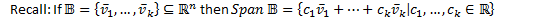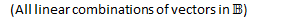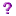Q: How do we find the smallest spanning set?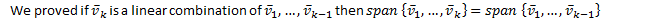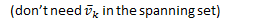Definition

A set of vectors is linearly dependent if one vector in the set is a linear combination of the remaining vectors.

Otherwise, the set of vectors is linearly independent.Is there a nice mathematical expression to define linear dependence?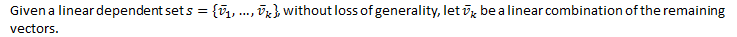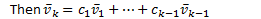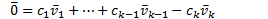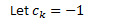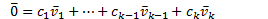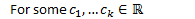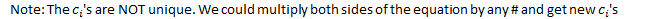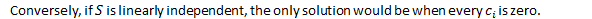Better Definition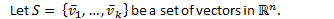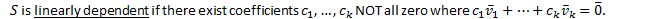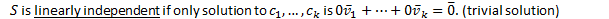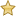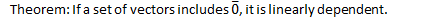Proof: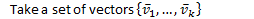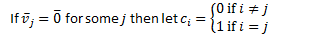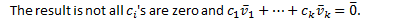Q.E.D.

Eg.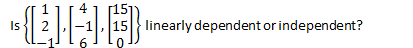Consider the equation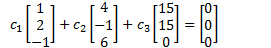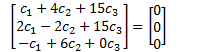We have 3 equations and 3 unknowns: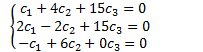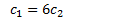Sub into equation 1: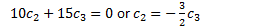Our set is linearly dependent.Note: In order for a spanning set to be as small as possible, it must be linearly independent.

Definition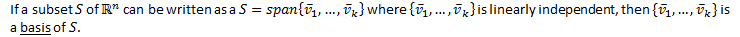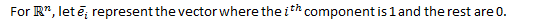Ex.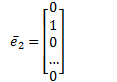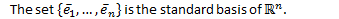Eg.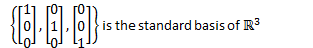Surfaces in Higher Dimensions

Definition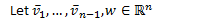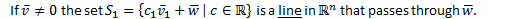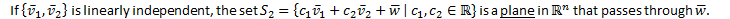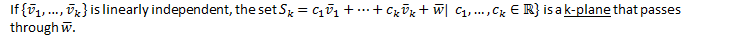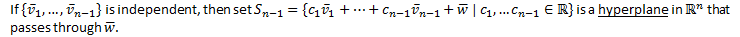Subspaces

For a set of vectors to be closed under linear combinations, we must be able to apply the operations of vector addition and scalar multiplication.

Definition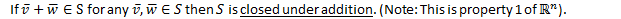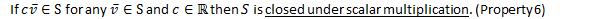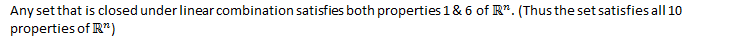Definition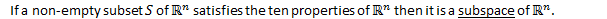Created by Tim Pei with Microsoft OneNote 2010
One place for all your notes and information